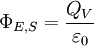# Gauss' Law and net charge

catie1981

## Homework Statement

The box-like Gaussian surface of the figure below encloses a net charge of +57.0ε0 C and lies in an electric field given by = [(10.3 + 2.4x)·i - 3.1·j + bz·k ] N/C, with x and z in meters and b a constant. The bottom face is in the xz plane; the top face is in the horizontal plane passing through y2 = 3.5 m. For x1 = 2.5 m, x2 = 4.7 m, z1 = 2.2 m, and z2 = 3.9 m, what is b?

Sorry that I have no figure here, hopefully you can imagine what the box looks like on the xyz plane.

I'm not sure!

## The Attempt at a Solution

I'd like to show one, but this whole thing has me stumped! I have no clue what they mean by 10.3+2.4x i or bz k, and I don't know how to use Gauss' Law for this. I'm sorry that this is all I have, but maybe if someone could get me started by explaining the E value first! And then which form(?) of Gauss' Law to use...(Will I be integrating or not??) Thanks in advance for any and all help.

Homework Helper
Ok so the form of the Electric field means that it changes as you move through space. For example at x = 0, the i component will be 10.3, but at x=1 the i component will be 12.7. Make sense?

Texag
Here's how you go about it. For a Gaussian surface, recall that the total flux out of any closed surface is known if you know the charge. You must also find the individual electric flux through each face of the cube by integrating. Then you have the total flux with "b" in it which is equal to what gauss's law gives you.

rootX
Relevant equation that you need:so, find area of each surface (6 surfaces of the box)

find flux through each (using area*E per to it)

use above equation

edit: oops wrong title lol

Homework Helper
@Rootx:

Not quite. Since the field varies with space, you will have to integrate over each surface.

rootX
@Rootx:

Not quite. Since the field varies with space, you will have to integrate over each surface.

oo yea, right!

catie1981
Relevant equation that you need:So, am I using this equation, and integrating? I am so confused as to what equation to use, since the book is a little unclear. I understand what the electric field form is saying now. And I'm assuming that to find the area I just use lxwxh=A corresponding to the x, y, and z values that I have. Although, since I'm still lost about my equation of choice, I don't know if I need that?

rootX
So, am I using this equation, and integrating? I am so confused as to what equation to use, since the book is a little unclear. I understand what the electric field form is saying now. And I'm assuming that to find the area I just use lxwxh=A corresponding to the x, y, and z values that I have. Although, since I'm still lost about my equation of choice, I don't know if I need that?

yes you need that one but before this you need one more that finds flux through each surface..

I think this is from halliday, and if I remember correctly, there is a simple example that has similar question.

no, that is not the area (l*w*h gives volume; area is a*b) it is

integral E.dA

and you would use x-z, xy and yz planes ..

You really really need to understand concepts (you should have solved simpler questions before this one).

catie1981
whoops, my bad about the are vs volume thing, not paying attention...
And I would like to understand concepts, but the book presents this all as math problems, spending very litte time on concepts. And, though my professor is good, I only have her during class time, as she doesn't have "office hours."
What is the Qv in the above equation, does that correspond to the +57.0ε0 C in the problem? (like q enclosed?) right now I'm trying to use the integral E*dA and determining the phi value, and will do that for 6 sides, but what will that do? Won't that only give me the value I already have (+57.0...)?

rootX
whoops, my bad about the are vs volume thing, not paying attention...
And I would like to understand concepts, but the book presents this all as math problems, spending very litte time on concepts. And, though my professor is good, I only have her during class time, as she doesn't have "office hours."
What is the Qv in the above equation, does that correspond to the +57.0ε0 C in the problem? (like q enclosed?) right now I'm trying to use the integral E*dA and determining the phi value, and will do that for 6 sides, but what will that do? Won't that only give me the value I already have (+57.0...)?

you add them up to get phi value in that equation (u add fluxes), and using that above equation (u know q, and u know the constant value), u will be able to find the unknown constant ..

And remember there is negative flux!

here's a short lecture on flux
http://iws.ccccd.edu/gsherman/Halliday%20-%20Chapter%2023.pdf [Broken]
you need to understand all of those to solve this problem (this involves all of those concepts)

Last edited by a moderator:
catie1981
when I do the integral for the right and left face I get something like this:

right face:
integral E*dA = integral (10.3+2.4x) dA
= 10.3+2.4(4.7) integral dA
= 21.58 * A (A=3.5*1.7=5.95)
= 128.4 Nm^2/C

left face:
-integral E*dA = -integral (10.3+2.4x)da
= -(10.3+2.4*2.5) integral dA
= -16.3 * A (A= 3.5 * 1.7=5.95)
= -96.99

is that right, and is that what the other faces will look like?

catie1981
whooops! it is! I got it solved! yeah! thanks!